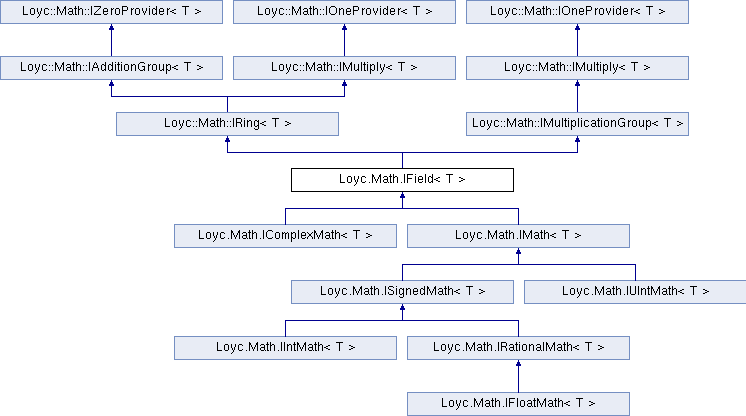Enhanced C# Language of your choice: library documentation
Loyc.Math.IField< T > Interface Template Reference

This defines a Field with the operations +,-,*,/ More...

Source file:
Inheritance diagram for Loyc.Math.IField< T >:## Remarks

This defines a Field with the operations +,-,*,/

Axioms that have to be satisified by the operations: The group axioms for + The group axioms for * Associativity: a * (b*c) = (a*b) * c Distributivity: a * (b+c) = (a*b) + (a*c) (a+b) * c = (a*c) + (b*c)Properties inherited from Loyc.Math.IZeroProvider< T >
Zero` [get]`
Returns the "zero" or additive identity of this type. More...Properties inherited from Loyc.Math.IOneProvider< T >
One` [get]`
Returns the "one" or identity value of this type. More...Public Member Functions inherited from Loyc.Math.IAdditionGroup< T >

Add (T a, T b, T c)

Sub (T a, T b)Public Member Functions inherited from Loyc.Math.IMultiply< T >
Mul (T a, T b)Public Member Functions inherited from Loyc.Math.IMultiplicationGroup< T >
Div (T a, T b)

Shl (T a, int amount)

Shr (T a, int amount)

MulDiv (T a, T mulBy, T divBy)